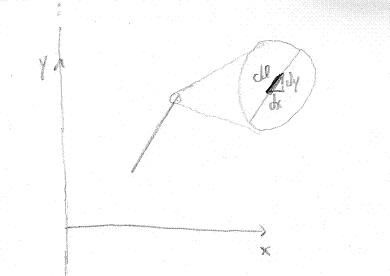# Thread: is my solution correct ..

1. ## is my solution correct ..

here is a question:
http://i25.tinypic.com/8xlcfl.gif
there is endless wire uniformly charged $\displaystyle \lambda=2*10^{-4}C/m$
and close to it located a wire with $\displaystyle l=0.12_m$
which has 30 degree angle between him and the endless wire,and it charged in uniformed way so that the total charge on it is $\displaystyle q=3*10^{-9}$
the distance of the finite wire and the infinite wire is 0.08 meters

A.find the total force acted on the finite wire.
B.find it when $\displaystyle \alpha=0$degrees and when $\displaystyle \alpha=90$degrees.

gaus law for infinite wire i presume its length is l and distance r.
$\displaystyle E2\pi r h=\frac{q}{\epsilon_0}\\$
$\displaystyle E=\frac{q}{\epsilon_0 2\pi r h}=\frac{\lambda h}{\epsilon_0 2\pi r h}=\frac{\lambda }{\epsilon_0 2\pi r }\\$
$\displaystyle \frac{q_2}{l_2}=\lambda_2\\$
$\displaystyle l_0=l_2\cos \alpha\\$
$\displaystyle \int_{0}^{l_0}Edl=\int_{0}^{l_0}\frac{\lambda }{\epsilon_0 2\pi r }dl$

i sum along the finite wire
but the ditance from the infinite changes too.
so it should be a double integral
but its not a 2d body

??

2. you are right, if its a 1 dimensional body you will need only 1 integral

but you also have to find out the relation of r with l.

hint:
if you draw a xy coordinate axis (parallel and perpendicular to the infinite wire), you can do it

then
$\displaystyle dl=\sqrt{dx^2+dy^2}$
$\displaystyle dl=\sqrt{(\frac{dx}{dx})^2+(\frac{dy}{dx})^2}dx$
$\displaystyle dl=\sqrt{1+(\frac{dy}{dx})^2}dx$
and you got a 1d integral

3. i cant use expression using dx and dy
because thus i wil have a double integral

i need to link l with r
r=0.08+(temporary hipotenuse)*cos alpha

i dont know how to express this temporary hipotenuse

4.Originally Posted by transgalactici cant use expression using dx and dy
because thus i wil have a double integral
no.
its not dx and dy. its dx and $\displaystyle \frac{dy}{dx}$.

Imagine these axis. y-axis parallel the infinite wire. x-axis perpendicular.
that way r will simply be x.

now you need to integrate dl, as you wrote in the end of your 1st post. but you need to write dl into a function of dx.notice the 90º angle triangle, you usenow you just need to find the wire equation
y=mx+b
calculate $\displaystyle \frac{dy}{dx}$ and you get dl in terms of dx.

Then substitute dl in your equation.
Actually your equation isnt entirely correct (Ithink)

F=E q
so you need to use
$\displaystyle dF=E \times dq$
$\displaystyle dF=E \times \lambda_2 \times dl$

5. i cant find
y=mx+b

m=dy/dx
but b cannot be found
i dont have enough data to know where the line crosses x axes

??

6.m is all you need
you only need dy/dx so find out m

7. my expestion is correct because its F=dq*E

and we substitute dl by an expression of dx
but i cant express dy only with dx
$\displaystyle \int_{0}^{l_0}Edl=\int_{0}^{xpretion}\frac{\lambda }{\epsilon_0 2\pi r }\sqrt{1+(\frac{dy}{dx})^2}dx$

8. i dont know how to express dy/dx using only dx

9. y=mx+b

if you derive that in relation to x

$\displaystyle \frac{dy}{dx}=m$

you look to the line and you see its 0.12m long and makes 30º with vertical, so
the x component of the line, $\displaystyle \Delta x$, is
$\displaystyle \Delta x=0.12 \times sin(30)=0.06$ m

the y component of the line, $\displaystyle \Delta y$, is
$\displaystyle \Delta y=0.12 \times cos(30)=0.06 \sqrt 3$ m

$\displaystyle m=\frac{\Delta y}{\Delta x}=\sqrt 3$

$\displaystyle \frac{dy}{dx}=\sqrt 3$

my expestion is correct because its F=dq*E

and we substitute dl by an expression of dx
but i cant express dy only with dxI don't understand.
if you say F=dq*E, why do you use in the integral E dl.
If you are calculating the Force you need to use E dq.

Note that nowhere in your expression the electric charge of the finite line is included. And the force clearly depends on that charge.

10. $\displaystyle E=\frac{\lambda }{\epsilon_0 2\pi r }\\$
$\displaystyle \int_{0}^{0.12}Edq=\int_{0}^{0.12}\frac{\lambda^2\ sqrt{1+3}dx }{\epsilon_0 2\pi r }=\int_{0.08}^{0.08+0.06 \sqrt 3}\frac{\lambda^22dx }{\epsilon_0 2\pi r }=\int_{0.08}^{0.08+0.06 \sqrt 3}\frac{\lambda^22dx }{\epsilon_0 2\pi x }$

correct?

11. it looks good

just use $\displaystyle \lambda_1 \lambda_2$ instead of $\displaystyle \lambda^2$, because the wires have different values.

and the integration limit should be
$\displaystyle \int_{0.08}^{0.08+0.06}$
because its $\displaystyle l \times sin(30)$

12. According to the figure, the distance from the center of the finite wire to the infinite wire is $\displaystyle x_0$. Integrate along the finite wire,

$\displaystyle F=\int_{-\tfrac{l}{2}}^{+\tfrac{l}{2}} \frac{\lambda}{2\pi \epsilon_0 (x_0+x\sin\alpha)} \ \frac{q}{l} \ dx = \frac{\lambda \ q}{2\pi \epsilon_0 l} \left(\frac{1}{\sin\alpha} \ln \frac{x_0+\frac{l}{2}\sin\alpha}{x_0-\frac{l}{2}\sin\alpha}\right)$

13. thanks

#### Search Tags

correct, solution